﻿ Big Crunch, Big Rip – or a Self-Similar Expansion Replenished by Dark Matter and Dark Energy?

### Big Crunch, Big Rip – or a Self-Similar Expansion Replenished by Dark Matter and Dark Energy?

Arne BergstromOPEN ACCESSPEER-REVIEWED

## Big Crunch, Big Rip – or a Self-Similar Expansion Replenished by Dark Matter and Dark Energy?

Arne BergstromB&E Scientific Ltd, Seaford BN25 4PA, United Kingdom

### Abstract

Our view of the universe, its origin and development has been a long, humbling sequence of misconceptions – not surprisingly so since this is perhaps the most fundamental question of all. This paper is a further attempt in trying to shed some light on this issue, and is based on the sole assumption that quantum propagation in space must obey a Lorentz-covariant continuity equation. This would seem to be perhaps the most basic assumption that can be made, and is shown to lead to an exponentially expanding universe, as observed, and also to force new quanta to be continuously created, which would thus explain the mysterious, dominating amounts of dark energy and dark matter observed in the universe. Mass-energy, diluted by the expansion, is thus in this way continuously being replenished. In the present paper, numerical simulations of this scenario are presented, which show a self-similar expansion, and in which new quanta are created all the time as the universe expands. The spatial distribution of the quanta involved is derived to be a Pareto distribution with an infinite mean free path, and which thus also continuously creates new self-similar clusters of quanta as embryos to new galaxies in regions far away from the original source.

### At a glance: Figures

12
Prev Next

• Bergstrom, Arne. "Big Crunch, Big Rip – or a Self-Similar Expansion Replenished by Dark Matter and Dark Energy?." International Journal of Physics 2.5 (2014): 146-150.
• Bergstrom, A. (2014). Big Crunch, Big Rip – or a Self-Similar Expansion Replenished by Dark Matter and Dark Energy?. International Journal of Physics, 2(5), 146-150.
• Bergstrom, Arne. "Big Crunch, Big Rip – or a Self-Similar Expansion Replenished by Dark Matter and Dark Energy?." International Journal of Physics 2, no. 5 (2014): 146-150.

 Import into BibTeX Import into EndNote Import into RefMan Import into RefWorks

### 1. Introduction

The structure and ultimate fate of the universe has occupied human thought ever since earliest times, and where we have now moved a long way from, e g, the ancient Babylonian view of the world as a giant oyster with water above and below . A fundamental problem in modern physics and astronomy is also to understand dark matter/energy: Why it is there at all, and why it is such a dominating part (95.1 % ) of the total mass of the universe.

The ultimate fate of the universe has in recent years been speculated to take many forms in between the two extremes of on one hand The Big Crunch , in which all matter of the universe finally implodes onto itself, and on the other hand The Big Rip , in which the observed accelerated expansion of the universe eventually leads to a universe where all galaxies surrounding us finally disappear over the horizon of observability, so that some hypothetical future observer could no longer see them.

The present paper aims at adding a new scenario to the discussion. From the sole assumption that quantum propagation in space should obey a Lorentz-covariant continuity equation – the Boltzmann transport equation – the observed exponential expansion of the universe can be stringently derived, and shown to involve a self-similar expansion and quantum creation. This requirement to obey a Lorentz-covariant continuity equation would seem to be the most basic assumption that can be made. This thus also leads to a scenario for the expanding universe in which new matter is being created all the time to form embryos to new galaxies, somewhat like how old and young trees are mixed in a forest.

As an unavoidable consequence of the requirement of relativistic covariance of the quantum transport, the picture below describes how the cosmological expansion is governed by a Pareto distribution and involves a quantum multiplication. The particular type of quanta involved in this process has a typical range of the size of galaxies (or perhaps rather that the size of galaxies is determined by this range). Within this range, these quanta will experience frequent “close” collisions, and are thereby subjected to a quantum doubling to preserve Lorentz covariance. This “close” dense web of such trajectories represents an increasing mass-energy concentration around each galaxy, which after sufficiently many collisions may by far exceed the “conventional” mass of the galaxy itself. This is here considered to be the mysterious “dark matter” observed to surround galaxies, and which accounts for 26.8 %  of the total mass-energy of the universe.

However, in addition to the trajectories formed by these “close” collisions, there is also another type of trajectories required by the properties of the Pareto distribution, namely extremely long trajectories due to the inverse-quadratic tail of the Pareto distribution (see Figure 1 and Figure 2). This tail thus populates the universe with “far” trajectories corresponding to the infinite mean free path that is also a characteristic of the Pareto distribution. This quantum population is also growing exponentially with time due to successive quantum doubling as the quanta travel through the universe. This extensive web of far trajectories is here considered to be the mysterious “dark energy” that is observed to fill the entire universe more or less homogeneously and accounting for 68.3 %  of its total mass-energy, thus together with dark matter allowing for only 4.9 % to be left as “normal matter”.

The first step in the study presented here was taken in a paper  in Nuovo Cimento already in 1975 – thus long before it was known that there was any dark matter/energy at all (cf, however, [6, 7]). This thus made it difficult to understand the full implications of the results obtained, including the quantum multiplication appearing in the derivation and the exponential expansion implicit in it. Then in 2011, in a paper published in Physica Scripta , the connection between the new astronomical observations and the previous theory was finally made, but only with regard to dark energy and the exponential expansion of the universe. Subsequent papers [9, 10] have then recently investigated various further aspects of this approach and also included dark matter into the picture , as will here also be discussed in Sec. 4 and illustrated in Figure 4A and Figure 4B below.

After a summary of the basic principles leading to the cosmological expansion and successive quantum doubling now following in Sec. 2 below, the self-similar nature of the expansion will be discussed in Secs. 3 and 4 below, after which the possibility of a Big Rip will be addressed in Sec. 5.

### 2. The General Continuity Equation

The exact development of a particle distribution as function of space and time can be summarised as follows.

Consider an ensemble of neutral particles moving with the velocity of light c through space. The time behaviour of such an ensemble is rigorously described by the Boltzmann transport equation  given as follows,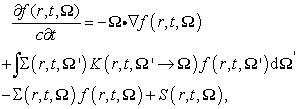(1)

where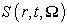is a source term, and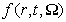is the angular flux of the particles in direction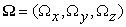at point = (x, y, z) and time t. Possible localised changes in the angular flux as the particles travel through space are described by the cross section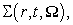and where the kernel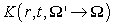then describes how particles may become scattered from direction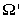to direction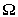, and/or partially absorbed or multiplied in the process. The Boltzmann transport equation has been used for over fifty years to calculate how, e g, gamma rays or neutrons propagate in nuclear reactors, nuclear weapons and radiation shields.

The cross section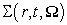historically describes the probability for the particles to react with a surrounding medium by absorption, scattering or multiplication (like in fission). However, in the following we will here let the cross section describe also the case when the particles themselves may experience spontaneous, localised duplications in their trajectories independently of the surrounding medium.

The total flux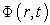is defined from the angular flux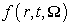as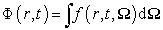(2)

Unlike the angular fluxin (1), the total fluxin (2) is normally not described by any exact continuity equation. However, for quanta being scattered isotropically in direction and homogeneously in space, the quantum propagation as described by the fluxin (2) can be derived [4, 5] rigorously from the Boltzmann transport equation (1) to take the form of the “telegrapher’s equation” [13, 14],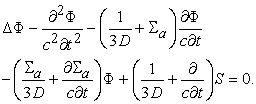(3)

where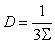(4)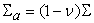(5)

with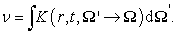(6)

Due to the third and fourth terms on the left-hand side of the telegrapher’s equation (3) above, this equation will not be Lorentz-covariant and it will also display dispersion – two properties that would make it incompatible with a wave equation. However, we note that the telegrapher’s equation above is compatible with a Lorentz-covariant and dispersion-free quantum propagation as described by a wave equation in the special case when the third and fourth terms in (3) vanish, i e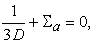(7)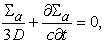(8)

which thus combine to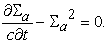(9)

The differential equation (9) has the following solution (R is an integration constant)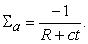(10)

From (4) and (7) we see that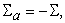and from (10) and (5), respectively, we thus get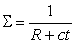(11)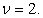(12)

The Lorentz-covariant and dispersion-free quantum transport derived above is thus a Markov process with a multiplication factor of 2, and a cumulative path length distribution function given by the following expression ,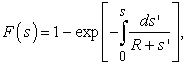(13)

which evaluates to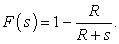(14)

i e a generalized Pareto distribution [16, 17], a distribution more commonly encountered in economics and sociology. The frequency distribution corresponding to the above cumulative path length distribution in (14) is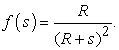(15)

A typical Pareto frequency distribution of this type is illustrated in Figure 1 and Figure 2.

The path-length distribution above is thus a rational function in contrast to the exponential path-length distribution normally encountered in particle transport theory. In particular – and quite different from normal particle propagation in a medium – the mean free path corresponding to the Pareto distribution above will be infinite,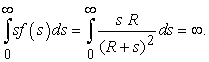(16)

From (16) we see that the asymptotic, inverse square law for large s in (15) thus means that there is a constant probability for a particle to reach any sufficiently large radial distance s from a collision point. The Pareto distribution thus provides a mechanism to populate far reaches of space with isolated new collision points, around each one of which new dense clusters of collision points will again form.

Figure 1. Pareto distribution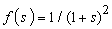compared to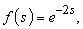coinciding for small s. Note the frequent occurrence of longer path lengths s in the Pareto distribution (cf Figure 2)
Figure 2. The Pareto distribution and negative exponential distribution in Figure 1 displayed in a loglog diagram in comparison with an inverse square distribution. The Pareto distribution agrees with a negative exponential for small s and with an inverse square law for large s

### 3. Self-similar Expansion of a Cluster

As discussed above, the propagation of a particle obeying the Boltzmann equation is described the Pareto distribution in (14) and (15). As illustrated in Figure 2, the Pareto distribution is characterised by two different regimes: I) a close field with an exponential decay of dense collisions, and II) a far field with equal probability of rare collisions for each far radial distance interval. In this section, the close-field I will be discussed, after which the far-field II will be discussed in Sec. 4 below.

Figure 3A and Figure 3B show the results of Monte Carlo simulations of the quantum distributions obtained when quanta are started at the origin and then followed in the close-field regime I for different numbers of successive collisions with the surrounding medium. Each such collision results in a doubling of the quantum at the collision, with each one of the two quanta following its own Pareto path in an arbitrary direction, independently of the original direction and of the direction of the other quantum. This type of assumed elastic scattering is thus modelled from quanta having low energy compared to the mass of the particles with which they collide.

Figure 3A. Simulations of the particle distribution as function of radial distance for a large number of particles, all started at the origin and followed for 6, 8, 12, and 14 generations, respectively, of particle doubling

This is thus a mechanism that after comparatively few generations of such doublings leads to very large numbers of particles (after only around 270 such doublings we will for example  reach the estimated number of particles {~1080} in the observable universe). In the present study, practical considerations with regard to statistics/storage capacity set the maximum number of generations to around 15.

Figure 3B. The four curves in Figure 3A scaled to the same peak value and peak position, illustrating that the curves for different generations have the same functional form, and that the particle distribution thus is self-similar

From Figure 3A and Figure 3B we see that the particle distributions obtained in such simulations in regime I close to the original source, and with different numbers of generations, are self-similar. This thus means that observers at different epochs will experience themselves to be surrounded by gross astronomical structures of the same general appearance regardless of epoch. The relationship with Big Rip scenarios will be discussed in Sec. 5 below.

### 4. Creation of Distant New Self-similar Clusters

Instead of quantum propagation in the close-field regime I as in Sec. 3 above, we now consider quantum propagation in the far-field regime II. We thus consider the situation when the Pareto distribution has given a new collision point far away from any previous collision points or trajectories, and now starts a new cluster.

Figure 4A shows a computer simulation, in which a trajectory is started at such a collision point at the origin and then followed through successive collisions as described by the Pareto distribution, and where each new collision becomes the starting point for two new trajectories, etc, as described above. In the case displayed in Figure 4A, the trajectory tree is followed through 14 generations.

In Figure 4A we clearly see the close-field of dense collisions clustering around the starting point. We also see how new such clusters are created further away from the original cluster, as illustrated by the daughter cluster in the upper left corner of Figure 4A, of which an enlargement is shown in Figure 4B. In particular, note the many open trajectories in Figure 4A without any collision point at the end, and thus leading out to collision points maybe extremely far away outside the volume shown and creating new dense clusters there.

In Figure 4B, note the trajectory in the lower left corner that just happens to pass the displayed volume without having any collision point inside the volume. This is an example of the situation in a region of the universe containing many generations of trajectories, and where then any region is normally heavily crisscrossed by a huge number of such trajectories from far away. These trajectories of type II far from their collision points then form an extended, more or less homogeneous fabric of mass-energy (“dark energy”) encompassing the universe, whereas the trajectories of type I discussed earlier form a dense mass-energy fabric (“dark matter”) localised in clusters around galaxies as described in Sec. 3 above.

Figure 4A. Spatial distribution of collision points (black) and trajectories (red) for 14 generations of particle doubling after starting a particle at the origin. Note the many open trajectories (“dark energy”) of regime-II-trajectories leading to collision points far away outside the volume shown, and creating new clusters much further away. The closely-knit regime-I-trajectories in the dense clusters of collision points are interpreted as “dark matter”
Figure 4B. Enlargement of the upper left corner of Figure 4A, showing a daughter cluster which in fact has just produced a second-generation daughter cluster to the right of the original daughter cluster

### 5. Self-similar Expansion and Big Rip

Somewhat paradoxically, the above analysis seems to give a possible explanation for the origin and nature of the strange dark matter/energy while leaving still unanswered the question of the origin of ordinary, familiar matter. In particular, the derivation in Sec. 2 above was specifically designed to allow the mechanism creating dark energy/matter not necessarily to depend on interactions with any surrounding medium. Yet it is not easy to see how the “close” quantum collisions resulting in dark matter concentrations around galaxies can be explained in any other way than that galaxies represent the essentially stationary matter against which the quanta representing dark matter collide.

It would seem that the relationship between ordinary matter and dark matter might be classified by the following alternatives:

Alternative α): Ordinary matter is primeval matter from the Big Bang. Dark matter, on the other hand, is created independently as a result Lorentz-covariance of the quantum propagation as discussed above.

Alternative β): As a more detailed variant of alternative α), dark matter duplication as discussed above involved particles with much heavier masses before the present epoch, but which due to successive degrading by particle duplication now have only very low masses left, and thus now appear only as diffuse clouds of low-mass particles around galaxies. Despite dark matter masses thus being continuously degraded at their duplications, the expansion of the ordinary matter causes the ratio of dark matter to ordinary matter to increase all the time, and dark matter/energy thus becoming a successively greater proportion of the total matter within the horizon of observability.

Alternative γ): Dark matter is being created all the time in the form of new clusters of successively greater amounts of solely dark matter. Eventually these clusters form galaxy-like structures in which their mutual gravitational attraction becomes so strong so as to form supermassive black holes in their centres. These black holes are thus formed exclusively from dark matter as it comes too close. This dark matter can then become rejuvenated in the form of relativistic jets of electrons and protons, as now occasionally are observed being emitted from normal galaxies. This may be a normal rejuvenating process in the life of galaxies , and can thus create pristine, normal matter also from dark matter.

Alternatives α) and β) above both lead to Big Rip scenarios. Alternative γ), although more complicated, might lead to development of galaxies with much longer life spans, and not necessarily involving a Big Rip as their final fate.

It does not at the present time seem possible to decide between the above alternatives. Alternative β) may possibly seem the most likely of the three scenarios. Alternative γ) may on the other hand perhaps seem attractive since it might permit universes with longer, non-trivial life-times, but it also seems more complicated and parameter-dependent. Nevertheless, it would be a challenge to see if it would be possible to find such “dark galaxies”, which would then manifest themselves essentially only by their gravitational distortion of light from sources behind them. If such distortions could be found with no apparent optical source in front causing them, then that could be an indication that alternative γ) might also possibly be a valid alternative.

### 6. Concluding Remarks

This communication discusses a model of the universe involving a self-similar expansion, with embryos to new galaxies being born all the time to replace ageing ones. One aspect of self-similarity is that it might allow for a much more permanent type of universe, thus removing the problem to explain why we are so fortunate to live in a universe not in the process of imploding onto itself, nor one in which there are no galaxies, maybe not even stars, to be seen within the horizon of our universe.

Just like quantum mechanics deals with a sub-microscopic world that runs counter to our intuition, biased as we are from experiences from the macroscopic world around us, so does cosmology deal with a super-astronomic world that might possibly be just as alien to our intuition. This stresses the need to show deep humility with regard to questions concerning the universe as a whole, its origin and ultimate fate. We should be well aware that our thoughts about these ultimate problems in cosmology may be but just more elaborate schemes of ignorance than the ancient Babylonian picture of the world as a giant oyster mentioned in the beginning. The real picture of the universe as a whole, its origin and development may still by far elude us. But unless we try to understand it anyway, we will get no further.

### References

  A. Koestler, The sleepwalkers: A history of man’s changing vision of the universe (Penguin, 1964; also in Kindle 2013).In article  http://en.wikipedia.org/wiki/Planck_(spacecraft) retrieved 2014-08-17.In article  G. Ellis, R. Maartens, and M. MacCallum, Relativistic Cosmology. (Cambridge, 2012), pp 146-47.In article CrossRef  R. Caldwell, M. Kamionkowski, and N. Weinberg, “Phantom Energy and Cosmic Doomsday”. Phys. Rev. Lett. 91, 7 (2003).In article CrossRef  A. Bergstrom, “Relativistic invariance and the expansion of the universe”, Nuovo Cimento 27B, 145-160 (1975).In article CrossRef  F. Zwicky, “Die Rotverschiebung von extragalaktischen Nebeln”, Helvetica Physica Acta 6, 110 (1933). F. Zwicky, “On the Masses of Nebulae and of Clusters of Nebulae”, Astrophys. J. 86, 217 (1937).In article CrossRef  A. Bergstrom, “Dark energy, exponential expansion, CMB, wave-particle duality all result from Lorentz-covariance of Boltzmann’s transport equation”, International Journal of Physics 2, 112-117 (2014).In article CrossRef  A. Bergstrom, “Lorentz-covariant quantum transport and the origin of dark energy”, Phys. Scr. 83, 055901 (2011).In article CrossRef  A. Bergstrom, “Is CMB just an observational effect of a universe in accelerated expansion?”, International Journal of Physics 1, 133-137 (2013).In article  A. Bergstrom, “An element in a paradigm shift? – Could Population II stars actually be younger than Population I stars?”, International Journal of Physics 2, 78-82 (2014).In article CrossRef  A. Bergstrom, “Why galaxies look like islands in the universe – and on the origin of dark matter and energy” (to be published).In article  A. M. Weinberg and E. P. Wigner, The Physical Theory of Neutron Chain Reactors (Univ. of Chicago Press, 1958), p. 232.In article  J. A. Stratton, Electromagnetic Theory (McGraw-Hill, 1941), p. 550.In article  A. M. Weinberg and E. P. Wigner, The Physical Theory of Neutron Chain Reactors (Univ. of Chicago Press, 1958), p. 235.In article  G. I. Bell and S. Glasstone, Nuclear Reactor Theory (Van Nostrand, 1970), p 54.In article  N. L. Johnson, S. Kotz, and N. Balakrishnan, Continuous Univariate Distributions. Vol 1, Ch 20, Sec 12 (Wiley, 1994).In article  http://en.wikipedia.org/wiki/Generalized_Pareto_Distribution retrieved 2014-08-17.In article